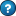Units: SI(mm) & US(in).Copy for Native Excel:
Purpose of calculation:
Calculate bending moments, shear, slope and deflection of a built in beam under a point load.
Calculation Reference
Roark's Formulas for Stress and Strain (7th Edition)
Table 8.1 case 1d - Left end fixed, right end fixed
Calculation Validation
Independent check on deflection checked at www.xcalcs.com
l = 5000 mm in
E = 210000 N/mm² lbf/in²
I = 1900000 mm⁴ in⁴
W = 5000 N lbf
a = 4000 mm in
Boundary Values
Vertical reaction at left end
RA = =(E14*(E11-E15)^2*(E11+2*E15))/(E11^3) N lbf
Moment reaction at left end
MA = =(-E14*E15*(E11-E15)^2)/(E11^2) Nmm lbfin
End slope at left end
Deflection at left end
yA = 0 mm in
Vertical reaction at right end
RB = =(E14*E15^2*(3*E11-2*E15))/(E11^3) N lbf
Moment reaction at right end
MB = =(-E14*E15^2*(E11-E15))/(E11^2) Nmm lbfin
End slope at right end
Deflection at right end
yB = 0 mm in
Selected maximum values of moments and deformations
Max + M = 2Wa²(l-a)²/l³ at x=a; max possible value = Wl/8 when a= l/2
Max -M=MA if a < l/2; max possible value = -0.1481Wl when a=1/3
Max y=-2W(l-a)²a³/3EI(l+2a)² at x=2al/(l+2a) when a > 1/2;
max possible value = -Wl3/192EI when x=a=1/2
Calculate values for any point along longitudinal axis of the beam
x = 3000 mm in x = 0 =AF55+\$E11/10 =AG55+\$E11/10 =AH55+\$E11/10 =AI55+\$E11/10 =AJ55+\$E11/10 =AK55+\$E11/10 =AL55+\$E11/10 =AM55+\$E11/10 =AN55+\$E11/10 =AO55+\$E11/10
Heavyside step function Heavyside step function
Hx = =IF(E55>\$E15,E55-\$E15,0) Hx = =IF(AF55>\$E15,AF55-\$E15,0) =IF(AG55>\$E15,AG55-\$E15,0) =IF(AH55>\$E15,AH55-\$E15,0) =IF(AI55>\$E15,AI55-\$E15,0) =IF(AJ55>\$E15,AJ55-\$E15,0) =IF(AK55>\$E15,AK55-\$E15,0) =IF(AL55>\$E15,AL55-\$E15,0) =IF(AM55>\$E15,AM55-\$E15,0) =IF(AN55>\$E15,AN55-\$E15,0) =IF(AO55>\$E15,AO55-\$E15,0) =IF(AP55>\$E15,AP55-\$E15,0)
Heavyside step function raised to the power 0 Heavyside step function raised to the power 0
Hx0 = =IF(E55>\$E15,1,0) Hx0 = =IF(AF55>\$E15,1,0) =IF(AG55>\$E15,1,0) =IF(AH55>\$E15,1,0) =IF(AI55>\$E15,1,0) =IF(AJ55>\$E15,1,0) =IF(AK55>\$E15,1,0) =IF(AL55>\$E15,1,0) =IF(AM55>\$E15,1,0) =IF(AN55>\$E15,1,0) =IF(AO55>\$E15,1,0) =IF(AP55>\$E15,1,0)
Transverse Shear Transverse Shear
V = =\$E21-\$E14*E59 N lbf V = =\$E21-\$E14*AF59 =\$E21-\$E14*AG59 =\$E21-\$E14*AH59 =\$E21-\$E14*AI59 =\$E21-\$E14*AJ59 =\$E21-\$E14*AK59 =\$E21-\$E14*AL59 =\$E21-\$E14*AM59 =\$E21-\$E14*AN59 =\$E21-\$E14*AO59 =\$E21-\$E14*AP59
Bending Moment Bending Moment
M = =\$E23+\$E21*E55-\$E14*E57 Nmm lbfin M = =\$E23+\$E21*AF55-\$E14*AF57 =\$E23+\$E21*AG55-\$E14*AG57 =\$E23+\$E21*AH55-\$E14*AH57 =\$E23+\$E21*AI55-\$E14*AI57 =\$E23+\$E21*AJ55-\$E14*AJ57 =\$E23+\$E21*AK55-\$E14*AK57 =\$E23+\$E21*AL55-\$E14*AL57 =\$E23+\$E21*AM55-\$E14*AM57 =\$E23+\$E21*AN55-\$E14*AN57 =\$E23+\$E21*AO55-\$E14*AO57 =\$E23+\$E21*AP55-\$E14*AP57
Slope Slope
θ = =\$E25+(\$E23*E55)/(\$E12*\$E13)+(\$E21*E55^2)/(2*\$E12*\$E13)-(\$E14*E57^2)/(2*\$E12*\$E13) rads rads θ = =\$E25+(\$E23*AF55)/(\$E12*\$E13)+(\$E21*AF55^2)/(2*\$E12*\$E13)-(\$E14*AF57^2)/(2*\$E12*\$E13) =\$E25+(\$E23*AG55)/(\$E12*\$E13)+(\$E21*AG55^2)/(2*\$E12*\$E13)-(\$E14*AG57^2)/(2*\$E12*\$E13) =\$E25+(\$E23*AH55)/(\$E12*\$E13)+(\$E21*AH55^2)/(2*\$E12*\$E13)-(\$E14*AH57^2)/(2*\$E12*\$E13) =\$E25+(\$E23*AI55)/(\$E12*\$E13)+(\$E21*AI55^2)/(2*\$E12*\$E13)-(\$E14*AI57^2)/(2*\$E12*\$E13) =\$E25+(\$E23*AJ55)/(\$E12*\$E13)+(\$E21*AJ55^2)/(2*\$E12*\$E13)-(\$E14*AJ57^2)/(2*\$E12*\$E13) =\$E25+(\$E23*AK55)/(\$E12*\$E13)+(\$E21*AK55^2)/(2*\$E12*\$E13)-(\$E14*AK57^2)/(2*\$E12*\$E13) =\$E25+(\$E23*AL55)/(\$E12*\$E13)+(\$E21*AL55^2)/(2*\$E12*\$E13)-(\$E14*AL57^2)/(2*\$E12*\$E13) =\$E25+(\$E23*AM55)/(\$E12*\$E13)+(\$E21*AM55^2)/(2*\$E12*\$E13)-(\$E14*AM57^2)/(2*\$E12*\$E13) =\$E25+(\$E23*AN55)/(\$E12*\$E13)+(\$E21*AN55^2)/(2*\$E12*\$E13)-(\$E14*AN57^2)/(2*\$E12*\$E13) =\$E25+(\$E23*AO55)/(\$E12*\$E13)+(\$E21*AO55^2)/(2*\$E12*\$E13)-(\$E14*AO57^2)/(2*\$E12*\$E13) =\$E25+(\$E23*AP55)/(\$E12*\$E13)+(\$E21*AP55^2)/(2*\$E12*\$E13)-(\$E14*AP57^2)/(2*\$E12*\$E13)
Deflection Deflection
y= =\$E27+\$E25*E55+(\$E23*E55^2)/(2*\$E12*\$E13)+(\$E21*E55^3)/(6*\$E12*\$E13)-(\$E14*E57^3)/(6*\$E12*\$E13) mm in y= =\$E27+\$E25*AF55+(\$E23*AF55^2)/(2*\$E12*\$E13)+(\$E21*AF55^3)/(6*\$E12*\$E13)-(\$E14*AF57^3)/(6*\$E12*\$E13) =\$E27+\$E25*AG55+(\$E23*AG55^2)/(2*\$E12*\$E13)+(\$E21*AG55^3)/(6*\$E12*\$E13)-(\$E14*AG57^3)/(6*\$E12*\$E13) =\$E27+\$E25*AH55+(\$E23*AH55^2)/(2*\$E12*\$E13)+(\$E21*AH55^3)/(6*\$E12*\$E13)-(\$E14*AH57^3)/(6*\$E12*\$E13) =\$E27+\$E25*AI55+(\$E23*AI55^2)/(2*\$E12*\$E13)+(\$E21*AI55^3)/(6*\$E12*\$E13)-(\$E14*AI57^3)/(6*\$E12*\$E13) =\$E27+\$E25*AJ55+(\$E23*AJ55^2)/(2*\$E12*\$E13)+(\$E21*AJ55^3)/(6*\$E12*\$E13)-(\$E14*AJ57^3)/(6*\$E12*\$E13) =\$E27+\$E25*AK55+(\$E23*AK55^2)/(2*\$E12*\$E13)+(\$E21*AK55^3)/(6*\$E12*\$E13)-(\$E14*AK57^3)/(6*\$E12*\$E13) =\$E27+\$E25*AL55+(\$E23*AL55^2)/(2*\$E12*\$E13)+(\$E21*AL55^3)/(6*\$E12*\$E13)-(\$E14*AL57^3)/(6*\$E12*\$E13) =\$E27+\$E25*AM55+(\$E23*AM55^2)/(2*\$E12*\$E13)+(\$E21*AM55^3)/(6*\$E12*\$E13)-(\$E14*AM57^3)/(6*\$E12*\$E13) =\$E27+\$E25*AN55+(\$E23*AN55^2)/(2*\$E12*\$E13)+(\$E21*AN55^3)/(6*\$E12*\$E13)-(\$E14*AN57^3)/(6*\$E12*\$E13) =\$E27+\$E25*AO55+(\$E23*AO55^2)/(2*\$E12*\$E13)+(\$E21*AO55^3)/(6*\$E12*\$E13)-(\$E14*AO57^3)/(6*\$E12*\$E13) =\$E27+\$E25*AP55+(\$E23*AP55^2)/(2*\$E12*\$E13)+(\$E21*AP55^3)/(6*\$E12*\$E13)-(\$E14*AP57^3)/(6*\$E12*\$E13)
Plot Deflection for 10 Points along the Length of the BeamCopy for XLC Enhanced Excel:
Purpose of calculation:
Calculate bending moments, shear, slope and deflection of a built in beam under a point load.
Calculation Reference
Roark's Formulas for Stress and Strain (7th Edition)
Table 8.1 case 1d - Left end fixed, right end fixed
Calculation Validation
Independent check on deflection checked at www.xcalcs.com
l = 5000 mm in
E = 210000 N/mm² lbf/in²
I = 1900000 mm⁴ in⁴
W = 5000 N lbf
a = 4000 mm in
Boundary Values
Vertical reaction at left end
RA = =OVD(E14*(E11-E15)^2*(E11+2*E15))/UND(E11^3) N lbf =EQS(E21,"Units= ; EqnPrefix=Eqn.; EqnNo= 0; Multiplication= 0; ShowWorking= 0; EqnStyle= 0; Eqp\$H\$25_0")
Moment reaction at left end
MA = =OVD(-E14*E15*(E11-E15)^2)/UND(E11^2) Nmm lbfin =EQS(E23,"Units= ; EqnPrefix=Eqn.; EqnNo= 0; Multiplication= 0; ShowWorking= 0; EqnStyle= 0; Eqp\$H\$27_0")
End slope at left end
Deflection at left end
yA = 0 mm in
Vertical reaction at right end
RB = =OVD(E14*E15^2*(3*E11-2*E15))/UND(E11^3) N lbf =EQS(E30,"Units= ; EqnPrefix=Eqn.; EqnNo= 0; Multiplication= 0; ShowWorking= 0; EqnStyle= 0; Eqp\$H\$34_0")
Moment reaction at right end
MB = =OVD(-E14*E15^2*(E11-E15))/UND(E11^2) Nmm lbfin =EQS(E32,"Units= ; EqnPrefix=Eqn.; EqnNo= 0; Multiplication= 0; ShowWorking= 0; EqnStyle= 0; Eqp\$H\$36_0")
End slope at right end
Deflection at right end
yB = 0 mm in
Selected maximum values of moments and deformations
Max + M = 2Wa²(l-a)²/l³ at x=a; max possible value = Wl/8 when a= l/2
Max -M=MA if a < l/2; max possible value = -0.1481Wl when a=1/3
Max y=-2W(l-a)²a³/3EI(l+2a)² at x=2al/(l+2a) when a > 1/2;
max possible value = -Wl3/192EI when x=a=1/2
Calculate values for any point along longitudinal axis of the beam
x = 3000 mm in x = 0 =AF55+\$E11/10 =AG55+\$E11/10 =AH55+\$E11/10 =AI55+\$E11/10 =AJ55+\$E11/10 =AK55+\$E11/10 =AL55+\$E11/10 =AM55+\$E11/10 =AN55+\$E11/10 =AO55+\$E11/10
Heavyside step function Heavyside step function
Hx = =IF(E55>\$E15,E55-\$E15,0) =EQS(E57,"Units= ; EqnPrefix=Eqn.; EqnNo= 0; Multiplication= 0; ShowWorking= 0; EqnStyle= 0; Eqp\$H\$61_0") Hx = =IF(AF55>\$E15,AF55-\$E15,0) =IF(AG55>\$E15,AG55-\$E15,0) =IF(AH55>\$E15,AH55-\$E15,0) =IF(AI55>\$E15,AI55-\$E15,0) =IF(AJ55>\$E15,AJ55-\$E15,0) =IF(AK55>\$E15,AK55-\$E15,0) =IF(AL55>\$E15,AL55-\$E15,0) =IF(AM55>\$E15,AM55-\$E15,0) =IF(AN55>\$E15,AN55-\$E15,0) =IF(AO55>\$E15,AO55-\$E15,0) =IF(AP55>\$E15,AP55-\$E15,0)
Heavyside step function raised to the power 0 Heavyside step function raised to the power 0
Hx0 = =IF(E55>\$E15,1,0) =EQS(E59,"Units= ; EqnPrefix=Eqn.; EqnNo= 0; Multiplication= 0; ShowWorking= 0; EqnStyle= 0; Eqp\$H\$63_0") Hx0 = =IF(AF55>\$E15,1,0) =IF(AG55>\$E15,1,0) =IF(AH55>\$E15,1,0) =IF(AI55>\$E15,1,0) =IF(AJ55>\$E15,1,0) =IF(AK55>\$E15,1,0) =IF(AL55>\$E15,1,0) =IF(AM55>\$E15,1,0) =IF(AN55>\$E15,1,0) =IF(AO55>\$E15,1,0) =IF(AP55>\$E15,1,0)
Transverse Shear Transverse Shear
V = =\$E21-\$E14*E59 N lbf =EQS(E61,"Units= ; EqnPrefix=Eqn.; EqnNo= 0; Multiplication= 0; ShowWorking= 0; EqnStyle= 0; Eqp\$H\$65_0") V = =\$E21-\$E14*AF59 =\$E21-\$E14*AG59 =\$E21-\$E14*AH59 =\$E21-\$E14*AI59 =\$E21-\$E14*AJ59 =\$E21-\$E14*AK59 =\$E21-\$E14*AL59 =\$E21-\$E14*AM59 =\$E21-\$E14*AN59 =\$E21-\$E14*AO59 =\$E21-\$E14*AP59
Bending Moment Bending Moment
M = =\$E23+\$E21*E55-\$E14*E57 Nmm lbfin =EQS(E63,"Units= ; EqnPrefix=Eqn.; EqnNo= 0; Multiplication= 0; ShowWorking= 0; EqnStyle= 0; Eqp\$H\$67_0") M = =\$E23+\$E21*AF55-\$E14*AF57 =\$E23+\$E21*AG55-\$E14*AG57 =\$E23+\$E21*AH55-\$E14*AH57 =\$E23+\$E21*AI55-\$E14*AI57 =\$E23+\$E21*AJ55-\$E14*AJ57 =\$E23+\$E21*AK55-\$E14*AK57 =\$E23+\$E21*AL55-\$E14*AL57 =\$E23+\$E21*AM55-\$E14*AM57 =\$E23+\$E21*AN55-\$E14*AN57 =\$E23+\$E21*AO55-\$E14*AO57 =\$E23+\$E21*AP55-\$E14*AP57
Slope Slope
θ = =\$E25+OVD(\$E23*E55)/UND(\$E12*\$E13)+OVD(\$E21*E55^2)/UND(2*\$E12*\$E13)-OVD(\$E14*E57^2)/UND(2*\$E12*\$E13) rads rads =EQS(E65,"Units= ; EqnPrefix=Eqn.; EqnNo= 0; Multiplication= 0; ShowWorking= 0; EqnStyle= 0; Eqp\$H\$69_0") θ = =\$E25+OVD(\$E23*AF55)/UND(\$E12*\$E13)+OVD(\$E21*AF55^2)/UND(2*\$E12*\$E13)-OVD(\$E14*AF57^2)/UND(2*\$E12*\$E13) =\$E25+OVD(\$E23*AG55)/UND(\$E12*\$E13)+OVD(\$E21*AG55^2)/UND(2*\$E12*\$E13)-OVD(\$E14*AG57^2)/UND(2*\$E12*\$E13) =\$E25+OVD(\$E23*AH55)/UND(\$E12*\$E13)+OVD(\$E21*AH55^2)/UND(2*\$E12*\$E13)-OVD(\$E14*AH57^2)/UND(2*\$E12*\$E13) =\$E25+OVD(\$E23*AI55)/UND(\$E12*\$E13)+OVD(\$E21*AI55^2)/UND(2*\$E12*\$E13)-OVD(\$E14*AI57^2)/UND(2*\$E12*\$E13) =\$E25+OVD(\$E23*AJ55)/UND(\$E12*\$E13)+OVD(\$E21*AJ55^2)/UND(2*\$E12*\$E13)-OVD(\$E14*AJ57^2)/UND(2*\$E12*\$E13) =\$E25+OVD(\$E23*AK55)/UND(\$E12*\$E13)+OVD(\$E21*AK55^2)/UND(2*\$E12*\$E13)-OVD(\$E14*AK57^2)/UND(2*\$E12*\$E13) =\$E25+OVD(\$E23*AL55)/UND(\$E12*\$E13)+OVD(\$E21*AL55^2)/UND(2*\$E12*\$E13)-OVD(\$E14*AL57^2)/UND(2*\$E12*\$E13) =\$E25+OVD(\$E23*AM55)/UND(\$E12*\$E13)+OVD(\$E21*AM55^2)/UND(2*\$E12*\$E13)-OVD(\$E14*AM57^2)/UND(2*\$E12*\$E13) =\$E25+OVD(\$E23*AN55)/UND(\$E12*\$E13)+OVD(\$E21*AN55^2)/UND(2*\$E12*\$E13)-OVD(\$E14*AN57^2)/UND(2*\$E12*\$E13) =\$E25+OVD(\$E23*AO55)/UND(\$E12*\$E13)+OVD(\$E21*AO55^2)/UND(2*\$E12*\$E13)-OVD(\$E14*AO57^2)/UND(2*\$E12*\$E13) =\$E25+OVD(\$E23*AP55)/UND(\$E12*\$E13)+OVD(\$E21*AP55^2)/UND(2*\$E12*\$E13)-OVD(\$E14*AP57^2)/UND(2*\$E12*\$E13)
Deflection Deflection
y= =\$E27+\$E25*E55+OVD(\$E23*E55^2)/UND(2*\$E12*\$E13)+OVD(\$E21*E55^3)/UND(6*\$E12*\$E13)-OVD(\$E14*E57^3)/UND(6*\$E12*\$E13) mm in =EQS(E67,"Units= ; EqnPrefix=Eqn.; EqnNo= 0; Multiplication= 0; ShowWorking= 0; EqnStyle= 0; Eqp\$H\$71_0") y= =\$E27+\$E25*AF55+OVD(\$E23*AF55^2)/UND(2*\$E12*\$E13)+OVD(\$E21*AF55^3)/UND(6*\$E12*\$E13)-OVD(\$E14*AF57^3)/UND(6*\$E12*\$E13) =\$E27+\$E25*AG55+OVD(\$E23*AG55^2)/UND(2*\$E12*\$E13)+OVD(\$E21*AG55^3)/UND(6*\$E12*\$E13)-OVD(\$E14*AG57^3)/UND(6*\$E12*\$E13) =\$E27+\$E25*AH55+OVD(\$E23*AH55^2)/UND(2*\$E12*\$E13)+OVD(\$E21*AH55^3)/UND(6*\$E12*\$E13)-OVD(\$E14*AH57^3)/UND(6*\$E12*\$E13) =\$E27+\$E25*AI55+OVD(\$E23*AI55^2)/UND(2*\$E12*\$E13)+OVD(\$E21*AI55^3)/UND(6*\$E12*\$E13)-OVD(\$E14*AI57^3)/UND(6*\$E12*\$E13) =\$E27+\$E25*AJ55+OVD(\$E23*AJ55^2)/UND(2*\$E12*\$E13)+OVD(\$E21*AJ55^3)/UND(6*\$E12*\$E13)-OVD(\$E14*AJ57^3)/UND(6*\$E12*\$E13) =\$E27+\$E25*AK55+OVD(\$E23*AK55^2)/UND(2*\$E12*\$E13)+OVD(\$E21*AK55^3)/UND(6*\$E12*\$E13)-OVD(\$E14*AK57^3)/UND(6*\$E12*\$E13) =\$E27+\$E25*AL55+OVD(\$E23*AL55^2)/UND(2*\$E12*\$E13)+OVD(\$E21*AL55^3)/UND(6*\$E12*\$E13)-OVD(\$E14*AL57^3)/UND(6*\$E12*\$E13) =\$E27+\$E25*AM55+OVD(\$E23*AM55^2)/UND(2*\$E12*\$E13)+OVD(\$E21*AM55^3)/UND(6*\$E12*\$E13)-OVD(\$E14*AM57^3)/UND(6*\$E12*\$E13) =\$E27+\$E25*AN55+OVD(\$E23*AN55^2)/UND(2*\$E12*\$E13)+OVD(\$E21*AN55^3)/UND(6*\$E12*\$E13)-OVD(\$E14*AN57^3)/UND(6*\$E12*\$E13) =\$E27+\$E25*AO55+OVD(\$E23*AO55^2)/UND(2*\$E12*\$E13)+OVD(\$E21*AO55^3)/UND(6*\$E12*\$E13)-OVD(\$E14*AO57^3)/UND(6*\$E12*\$E13) =\$E27+\$E25*AP55+OVD(\$E23*AP55^2)/UND(2*\$E12*\$E13)+OVD(\$E21*AP55^3)/UND(6*\$E12*\$E13)-OVD(\$E14*AP57^3)/UND(6*\$E12*\$E13)
Plot Deflection for 10 Points along the Length of the BeamInclude in Your Website: Taken from the ExcelCalcs.com website.